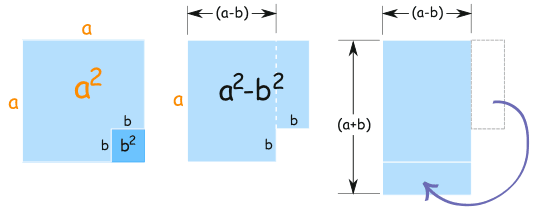# 特殊二项式乘积

## 二项式二项式例子

## 乘积

（乘）积 是乘法的结果。

(a+b)(a−b) 的意思是 (a+b) 乘以 (a−b)。我们在这里会时常用这个式子！

## 一、二项式自乘

(a+b)2 = (a+b)(a+b) = ……？

(a+b)2 = a2 + 2ab + b2## 二、减乘以减

(a−b)2 = (a−b)(a−b) = ……？

(a−b)2 = a2 − 2ab + b2

## 三、 加乘以减

(a+b)(a−b) = ……？

(a+b)(a−b) = a2 − b2a2 − b2      等于       (a+b)(a−b)

(a−b)(a+b) = a2 − b2

## 三个情况：

 (a+b)2 = a2 + 2ab + b2 } "完全平方三项式" (a−b)2 = a2 − 2ab + b2 (a+b)(a−b) = a2 − b2 "平方差"

## 使用

(y+1)2 = (y)2 + 2(y)(1) + (1)2 = y2 + 2y + 1

(3x−4)2 = (3x)2 − 2(3x)(4) + (4)2 = 9x2 − 24x + 16

(a+b)(a−b) = a2 − b2

(4y+2)(4y−2) = (4y)2 − (2)2 = 16y2 − 4

……看上去像个平方差……对不对？

4x2(2x)29(3)2，所以：

4x2 − 9 = (2x)2 − (3)2

(a+b)(a−b) = a2 − b2

(2x+3)(2x−3) = (2x)2 v (3)2 = 4x2 − 9